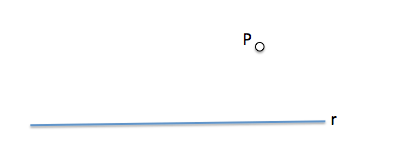# Arco able on a segment : Example [I]Applications in geometry able arc angle of a segment given are many and varied:

From the proof of a theorem, the intermediate solution of a problem or direct application in a case, we see repeated this construction generalized form.

Here is a simple example that directly apply this construction and conceptual but, to obtain the final solution, have to perform additional operations.

The arc able es el locus of the points from which a segment AB is "seen" with the same angle; namely, the locus of the vertices of the angles having the same amplitude and cover the same segment.(W)

Assume the following statement:

Determine two lines that are based on a point P outside a line r, together form an angle “alfa” given to the straight line and cut according to a segment of length “L”.

The starting geometry for the problem we see in the following image.Point “P” is outside the line “r”. The solution should be allowed to find two points, And the B, on the line “r” so as to determine the length of a segment “L” requested.We therefore an arch elements capable: An angular segment and a condition.

Could you solve this exercise?

The solution can be found at the following link

Solución

Metric geometry